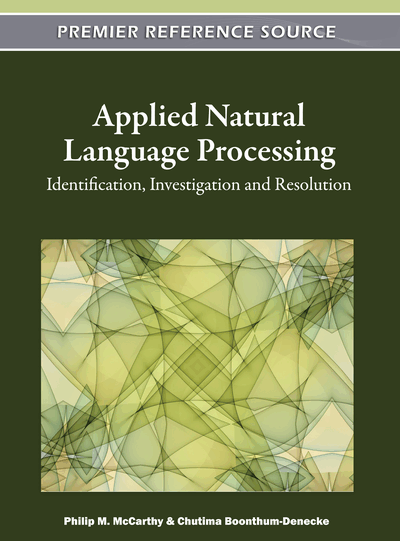# HiDEx: The High Dimensional Explorer

Cyrus Shaoul (University of Alberta, Canada) and Chris Westbury (University of Alberta, Canada)
Copyright: © 2012 |Pages: 17
DOI: 10.4018/978-1-60960-741-8.ch013

## Abstract

HAL (Hyperspace Analog to Language) is a high-dimensional model of semantic space that uses the global co-occurrence frequency of words in a large corpus of text as the basis for a representation of semantic memory. In the original HAL model, many parameters were set without any a priori rationale. In this chapter we describe a new computer application called the High Dimensional Explorer (HiDEx) that makes it possible to systematically alter the values of the model’s parameters and thereby to examine their effect on the co-occurrence matrix that instantiates the model. New parameter sets give us measures of semantic density that improve the model’s ability to predict behavioral measures. Implications for such models are discussed.
Chapter Preview
Top

## Introduction To Hal

We begin with a brief overview of the original HAL model (Burgess, 1998; Burgess & Lund, 2000).

HAL uses word co-occurrence to build an abstract data representation called a vector space that contains contextual information for every word in a specified dictionary. A vector space is a geometric representation of data that has an ordered set of N numbers associated with each point in an N-dimensional space. For example, any location on the earth’s surface can be specified by an ordered set of two numbers (a vector of length two), consisting of the location’s latitude and longitude. If we wanted to specify a point off the earth’s surface, we would need to add a third number specifying height above or below the surface, requiring us to specify each point with an ordered set of three numbers (a vector of length three). Just as in these examples, in higher dimensions each ordered set of numbers, or vector, defines a point’s location in a space.

Conceptually, a vector space can have dimensionality of any size. Each number in a vector simply specifies one quantitative attribute or characteristic of the point in its space. While locations on earth can be fully described with a set of three values, many things require more than three values to define them. We might imagine that instead of simply specifying a point on earth, we wanted to also specify the point’s color, temperature, and noise level, so that we would now need a six dimensional space, and therefore vectors of length six to describe every point. The vector space of HAL is made up of vectors for each word in the language. Because HAL includes information about every word’s co-occurrence relation to every word in the language (including itself), each vector in HAL has an entry for every word in the language. In the original HAL model, these vectors had more than 100,000 dimensions. Each entry in a HAL vector is a count of the number of times another word co-occurred with the vector’s word in a large text corpus, weighted by a factor that specifies how distant the two words were. Words can co-occur when they are adjacent, or when they are separated by a number of intervening words. The maximum distance between words considered to co-occur is called the window size. Window size is one of the free parameters in the HAL model. In the original model, words were considered to have co-occurred if they occurred within ten words of each other, in either direction.

The words in a target word’s co-occurrence window are weighted according to their proximity to that target word using a weighting function. The original HAL model used a linear weighting function, called a linear ramp, as a multiplier to give more weight to the words that co-occurred closer to the center of the window. Words that occurred on either side of the center word of the window were assigned ten co-occurrence points. The center word’s next neighbors were assigned nine co-occurrence points, and so on, down a single point for a word that occurred ten words away from the center word (see Figure 1, for an explanatory example with a window size of five).

## Complete Chapter List

Search this Book:
Reset